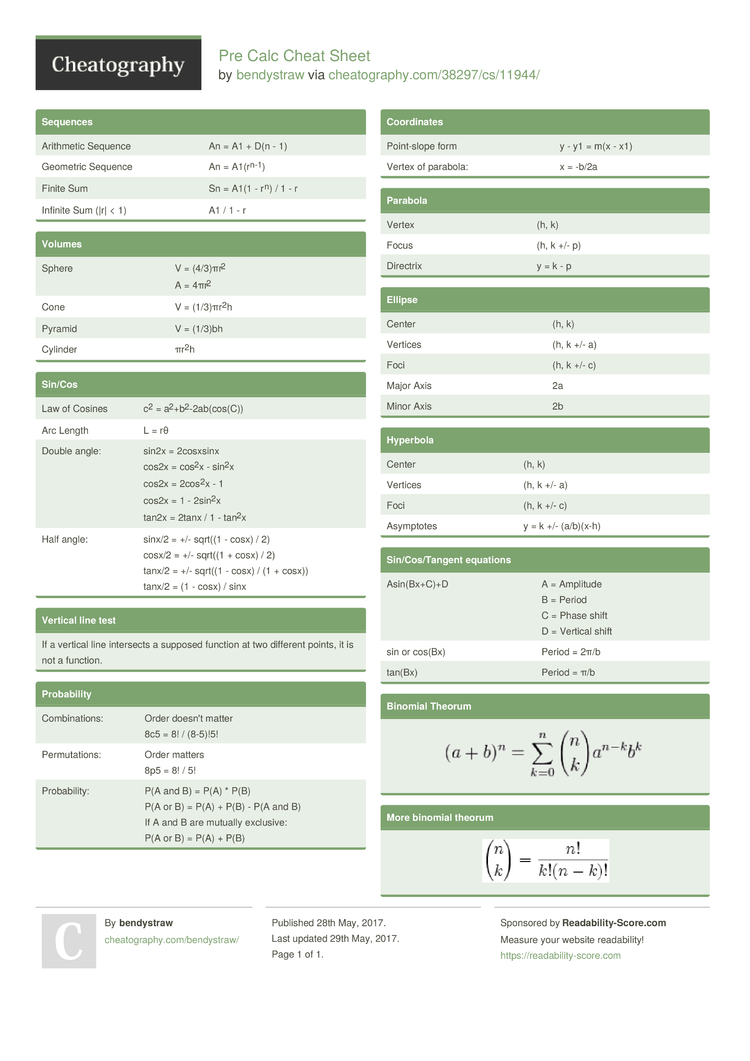# Pre Calc Cheat Sheet by bendystraw

### Sequences

 Arithmetic Sequence An = A1 + D(n - 1) Geometric Sequence An = A1(rn-1) Finite Sum Sn = A1(1 - rn) / 1 - r Infinite Sum (|r| < 1) A1 / 1 - r

### Volumes

 Sphere V = (4/3)πr2A = 4πr2 Cone V = (1/3)π­r2h Pyramid V = (1/3)bh Cylinder πr2h

### Sin/Cos

 Law of Cosines c2 = a2+b­2­-2a­b(c­os(C)) Arc Length L = rθ Double angle: sin2x = 2cosxsinxcos2x = cos2x - sin2xcos2x = 2cos2x - 1cos2x = 1 - 2sin2xtan2x = 2tanx / 1 - tan2x Half angle: sinx/2 = +/- sqrt((1 - cosx) / 2)cosx/2 = +/- sqrt((1 + cosx) / 2)tanx/2 = +/- sqrt((1 - cosx) / (1 + cosx))tanx/2 = (1 - cosx) / sinx

### Vertical line test

 If a vertical line intersects a supposed function at two different points, it is not a function.

### Probab­ility

 Combin­ations: Order doesn't matter8c5 = 8! / (8-5)!5! Permut­ations: Order matters8p5 = 8! / 5! Probab­ility: P(A and B) = P(A) * P(B)P(A or B) = P(A) + P(B) - P(A and B)If A and B are mutually exclusive:P(A or B) = P(A) + P(B)

### Coordi­nates

 Point-­slope form y - y1 = m(x - x1) Vertex of parabola: x = -b/2a

### Parabola

 Vertex (h, k) Focus (h, k +/- p) Directrix y = k - p

### Ellipse

 Center (h, k) Vertices (h, k +/- a) Foci (h, k +/- c) Major Axis 2a Minor Axis 2b

### Hyperbola

 Center (h, k) Vertices (h, k +/- a) Foci (h, k +/- c) Asymptotes y = k +/- (a/b)(x-h)

### Sin/Co­s/T­angent equations

 Asin(B­x+C)+D A = AmplitudeB = PeriodC = Phase shiftD = Vertical shift sin or cos(Bx) Period = 2π/b tan(Bx) Period = π/b

### Binomial Theorum### More binomial theorum1 Page
//media.cheatography.com/storage/thumb/bendystraw_pre-calc.750.jpg

PDF (recommended)